Next: Learning algorithm for the Up: Optimising the cost function Previous: Finding optimal for Dirichlet   Contents

### Finding optimal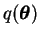for Gaussian parameters

As an example of Gaussian parameters we shall consider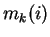and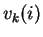. All the others are handled in essentially the same way except that there are no weights needed for different states.

To simplify the notation, all the indices fromandare dropped out for the remainder of this section. The relevant terms of the cost function are now, up to an additive constant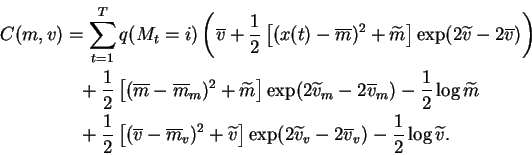(6.18)

Let us denote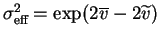,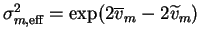and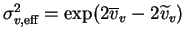.

The derivative of this expression with respect to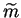is easy to evaluate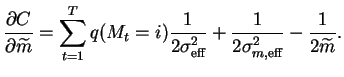(6.19)

Setting this to zero gives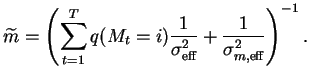(6.20)

The derivative with respect to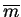is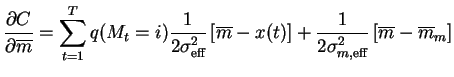(6.21)

which has a zero at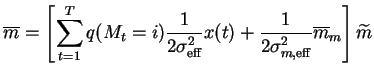(6.22)

whereis given by Equation (6.20).

The solutions for parameters of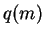are exact. The true posterior for these parameters is also Gaussian so the approximation is equal to it. This is not the case for the parameters of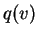. The true posterior for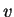is not Gaussian. The best Gaussian approximation with respect to the chosen criterion can still be found by solving the zero of the derivative of the cost function with respect to the parameters of. This is done using Newton's iteration.

The derivatives with respect to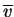and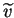are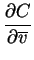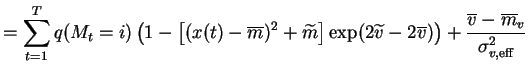(6.23)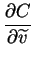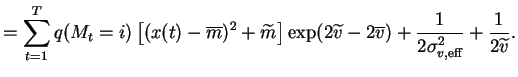(6.24)

These are set to zero and solved with Newton's iteration.Next: Learning algorithm for the Up: Optimising the cost function Previous: Finding optimal for Dirichlet   Contents
Antti Honkela 2001-05-30